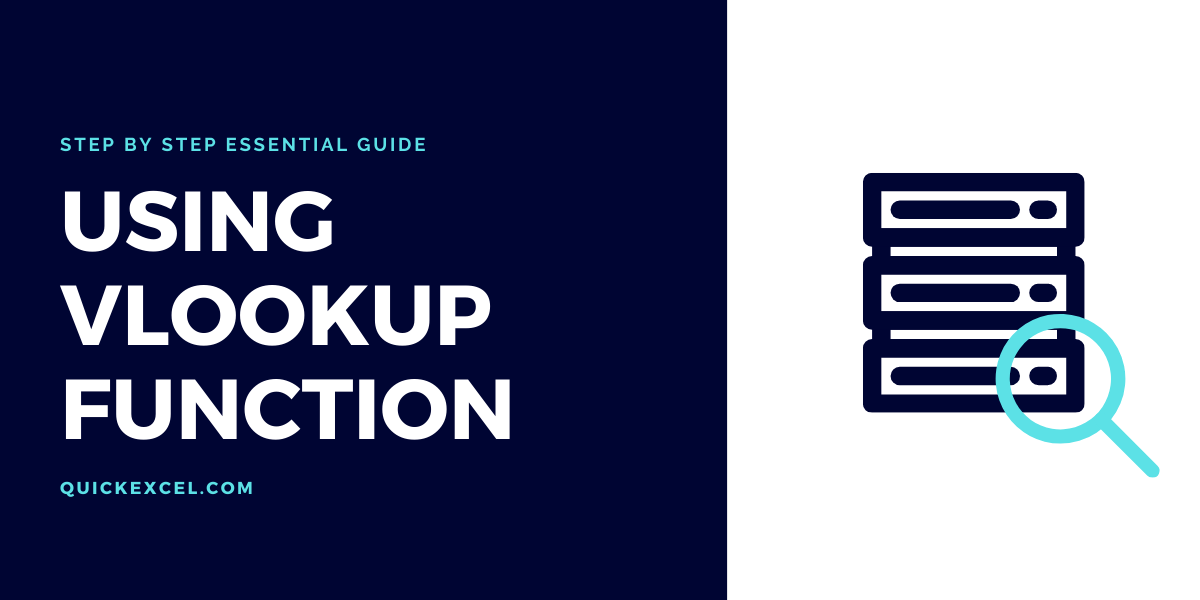# What is VLOOKUP and How to Use VLOOKUP in Excel?## VLOOKUP function in Excel

VLOOKUP is also known as Vertical Lookup is an important tool in Microsoft Excel that is generally used to lookup values in a vertical database.

It can help you save time and effort by automatically looking up the details for you from a large vertical database instead of you having to manually do the task.

VLOOKUP function is one that is applied to a database that requires the presence of the value you are looking up, its result, column numbers and specify whether the result should be an exact or approximate match to execute these tasks.

### Benefits of the VLOOKUP function

• Saves time and lessened manual efforts.
• Looks up values and results in a vertical database.
• It is swift and automatic.
• Easy to operate.

## How to use VLOOKUP function in Excel?

Let’s get started with this step-by-step guide learning to use the VLOOKUP function in Excel to be able to automatically lookup values in a vertical database.

### Prepare a vertical database

First, we need to prepare a vertical tabular database to be able to look up values in it via the VLOOKUP function. Let us take a sample database below to proceed further.

Recommended read: How to Calculate Age in Excel?

### Applying VLOOKUP in Excel

The goal is to be able to look up complete employee details from this database by only providing their ID numbers. Let’s apply the function and get started.

• Reserve a cell to input the ID numbers to be used while lookup.
• Type ID NUMBER in a cell below the database.
• Shift to the adjacent cell and start typing the formula.
• Type =VLOOKUP(
• The first argument you will be asked for is a lookup value which simply means the value for you want to use to pull the results. Here, it is the cell below the database called ID NUMBER. This is because we want to look up values present in the database by providing an ID number which is also present in the database.
• Select ID NUMBER when asked for lookup value, like this.
• Put a comma to move to the next argument i.e., Table Array. Table array is the entire table or range from which you are looking up results.
• Select the entire database at the table array argument like this.
• Put a comma to move to the next argument i.e., Col index num. Col index num is the column number or the column in the database from which you are looking up results.
• Type the number of the column determining its position from left to right. Here we are referring to the NAME column which is the second column in the database. Type 2 for the second column.
• Put a comma and move to the next argument i.e., Range lookup. In this argument, you are supposed to mention whether you want the result to be an exact match or an approximate match.
• Type 0 or FALSE if you want an exact match.
• Type 1 or TRUE if you want an approximate match.
• Press ENTER to complete the function.
• You should be able to see the column name from your database in the cell you put the function in. This means your function has been applied correctly.
• Apply the function for every column you want to look up results for.
• We will now apply the function for all the columns to completely automate our database.
• Remember, you will always choose the lookup value as ID NUMBER below the database, for every new VLOOKUP function for a different column.

You can now see that the VLOOKUP function has been applied for all columns correctly.

### Using VLOOKUP to look up values in a database

Let’s get started with using the applied function in practice and check if VLOOKUP is able to fulfill our requirement of looking up employee details from this database.

• Type the ID Number of an employee from the data table you are looking up.
• For example, type 1400 and press ENTER.

You can see that the VLOOKUP function is able to look up results from the database for ID number 1400, who is an employee named Bentley, aged 23, working in the HR department. You can also view other details that you needed to be provided.

### How to Use VLOOKUP in Excel with Two Spreadsheets?

Let’s learn to use the VLOOKUP in Excel with two spreadsheets.

We will take the same database to lookup values in a different sheet. The database is present in a different sheet and we will look up its results in another sheet.

• Put the ID Numbers of the employees for whom you want to look up results in a different sheet.
• Type the VLOOKUP function as =VLOOKUP(
• Select the ID number for which you want to look up results.
• Put a comma to move to the next argument i.e., Table Array.
• Go to the sheet in which the database is present and select the entire database.
• Put a comma to move to the next argument i.e., Col index num.
• Enter the column number under which you are looking up. Let’s enter 2 for the second column.
• Put a comma to move to the next argument i.e., Range lookup.
• Type 0 or FALSE if you want an exact match.
• Type 1 or TRUE if you want an approximate match.
• Press ENTER to complete the function.

This is how your formula should look like before hitting ENTER.

You can now see the result looked up by the VLOOKUP function in a different sheet.